# Maximum Likelihood Estimation – finding the best Parametric Model

Consider the situation where a collection of data samples has been obtained. It is assumed that this data is drawn from a parametric model. The parameters of this model are unknown.

For example, the collection of data samples can be the heights of all the students in a class. It is assumed that this data fits a Gaussian distribution. A Gaussian distribution is defined by two parameters – the mean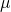and the standard deviation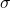. The goal is to find the best Gaussian distribution (defined by the parameters) that fits the data.

To find the best parameters that fit the data samples, the probability function of the parameters is computed using the given data. The goal of Maximum Likelihood Estimate is to find the parameter value(s) which maximizes this probability.

## Likelihood

The Likelihood is defined as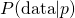. This is a function of both the data and the parameter(s)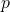. The likelihoodchanges as the parameter of interestchanges.

## Maximum Likelihood Estimate (MLE)

The maximum likelihood estimate (MLE) for the parameteris the value ofthat maximizes the likelihood. That is, the MLE is the value offor which the data is most likely.

The notation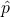is used for the MLE. It can be computed by taking the derivative of the likelihood function and setting it to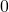.

## Example 1

A coin is flipped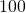times. Given that there were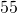heads, find the maximum likelihood estimate for the probabilityof heads on a single toss.

### Data

The data is the result of the experiment. In this case it is 55 heads.

### Parameter(s) of Interest

The value of the unknown parameterFor a given value of, the probability of gettingheads in this experiment is the binomial probability.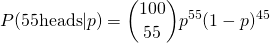This is read as “the probability ofheads given that the probability of heads on a single toss is.”

Setting the derivative to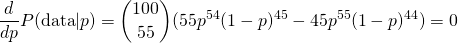Solving for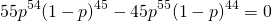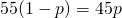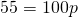Thus the MLE is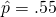## Log Likelihood

If is often easier to work with the natural log of the likelihood function. For short this is simply called the log likelihood. Since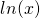is an increasing function, the maxima of the likelihood and log likelihood coincide.

## Example 2

Redoing Example 1 using log likelihood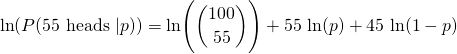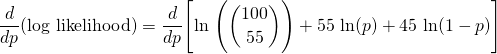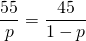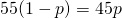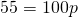Thus the MLE is## Example 3

Suppose that a particular gene occurs as one of two alleles (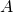and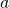), where allelehas frequency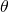in the population. That is, a random copy of the gene iswith probabilityandwith probability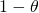. Since a diploid genotype consists of two genes, the probability of each genotype is given by

A test of random sample of people found that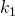are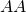,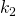are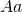, and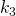are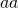. Find the MLE of.

### Dataare,are, andare.

### Parameter(s) of InterestThe Likelihood is given by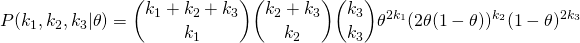The log Likelihood is given by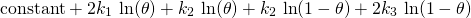Set the derivative equal to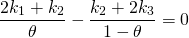Solving for,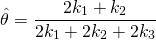which is simply the fraction ofalleles among all the genes in the sampled population.